## MP Board Class 7th Maths Solutions Chapter 5 Lines and Angles Ex 5.1

Question 1.
Find the complement of eachof the following angles: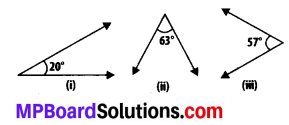Solution:
The sum of the measures of complementary angles is 90°.
(i) Complement of 20° = 90°- 20° = 70°
(ii) Complement of 63° = 90° – 63° = 27°
(iii) Complement of 57° = 90° – 57° = 33°

Question 2.
Find the supplement of each of the following angles: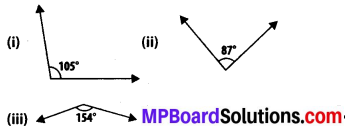Solution:
The sum of measures of supplementary angles is 180°.
(i) Supplement of 105° = 180° – 105° = 75°
(ii) Supplement of 87° = 180° – 87° = 93°
(iii) Supplement of 154° = 180° – 154° = 26°Question 3.
Identify which of the following pairs of angles are complementary and which are supplementary.
(i) 65°, 115°
(ii) 63°, 27°
(iii) 112°, 68°
(iv) 130°, 50°
(v) 45°, 45°
(vi) 80°, 10°
Solution:
The sum of the measures of complementary angles is 90° and that of supplementary angles is 180°.
(i) Sum of the measures of angles = 65° +115° = 180°
∴ These angles are supplementary angles.
(ii) Sum of the measures of angles = 63° + 27° = 90°
∴ These angles are complementary angles.
(iii) Sum of the measures of angles = 112° + 68° = 180°
∴ These angles are supplementary angles.
(iv) Sum of the measures of angles = 130° + 50° = 180°
∴ These angles are supplementary angles.
(v) Sum of the measures of angles = 45° + 45° = 90°
∴ These angles are complementary angles.
(vi) Sum of the measures of angles = 80°+ 10° =90°
∴ These angles are complementary angles.Question 4.
Find the angle which is equal to its complement.
Solution:
Let the angle be x.
Complement of this angle is also x.
∵ The sum of the measures of a pair of complementary angles is 90°.
∴ x + x = 90° ⇒ 2x = 90°

Question 5.
Find the angle which is equal to its supplement.
Solution:
Let the angle be x.
Supplement of this angle is also x.
∵ The sum of the measures of a pair of supplementary angles is 180°.
∴ x + x = 180°
⇒ 2x = 180° ⇒ x = 90°

Question 6.
In the given figure, ∠1 and ∠2 are supplementary angles. If ∠1 is decreased, what changes should take place in ∠2 so that both the angles still remain supplementary.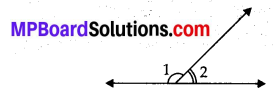Solution:
∠1 and ∠2 are supplementary angles.
If ∠1 is decreased, then ∠2 should be increased by the same measure so that the given pair of angles remains supplementary.

Question 7.
Can two angles be supplementary if both of them are:
(i) acute?
(ii) obtuse?
(iii) right?
Solution:
(i) No. Acute angle is always less than 90°. It can be observed that two angles, even of 89°, cannot add up to 180°. Therefore, two acute angles cannot form a supplementary.
(ii) No. Obtuse angle is always greater than 90°. It can be observed that two angles, even of 91°, will always add up to more than 180°. Therefore, two obtuse angles cannot form a supplementary.
(iii) Yes. Right angles are of 90° and 90° + 90° = 180°.
Therefore, two right angles form a supplementary angle together.Question 8.
An angle is greater than 45°. Is its complementary angle greater than 45° or equal to 45° or less than 45°?
Solution:
Let A and B are two angles making a complementary angle pair and A is greater than 45°.
A + B = 90°
⇒ B = 90° – A
Therefore, B will be less than 45°.

Question 9.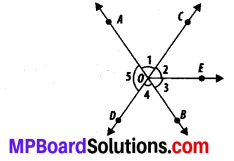(i) Is ∠1 adjacent to ∠2?
(ii) Is ∠AOC adjacent to ∠AOE?
(iii) Do ∠COE and ∠EOD form a linear pair?
(iv) Are ∠BOD and ∠DOA supplementary?
(v) Is ∠1 vertically opposite to ∠4?
(vi) What is the vertically opposite angle of ∠5?
Solution:
(i) Yes. Since, ∠1 and ∠2 have a common vertex O and a common arm OC. Also, their non-common arms OA and OE are on the opposite side of the common arm.
(ii) No. ∠AOC and ∠AOE have a common vertex O and also a common arm OA. But, their non-common arms OC and OE are on the same side of the common arm. Therefore, they are not adjacent to each other.
(iii) Yes. Since, ∠COE and ∠EOD have a common vertex O and a common arm OE. Also, their non-common arms, OC and OD, are opposite rays.
(iv) Yes. Since, ∠BOD and ∠DOA have a common vertex O and their non-common arms are opposite to each other.
(v) Yes. Since, ∠1 and ∠4 are formed due to the intersection of two straight lines, AB and CD.
(vi) ∠COB is the vertically opposite angle of ∠5 as they are formed due to the intersection of two straight lines, AB and CD.

Question 10.
Indicate which pairs of angles are:
(i) Vertically opposite angles.
(ii) Linear pairs.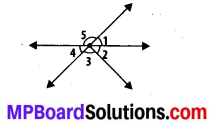Solution:
(i) ∠1 and ∠4, ∠5 and ∠2 + ∠3 are vertically opposite angles as they are formed due to the intersection of two straight lines.
(ii) ∠1 and ∠5, ∠5 and ∠4 are forming linear pairs as they have a common vertex and also, have non-common arms opposite to each other.Question 11.
In the following figure, is ∠1 adjacent to ∠2? Give reasons.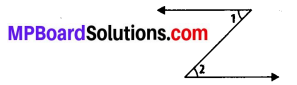Solution:
∠1 and ∠2 are not adjacent angles because their vertex is not common.

Question 12.
Find the values of the angles x, y and z in each of the following: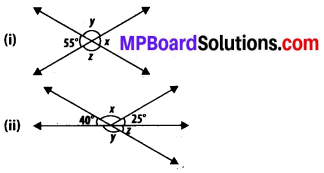Solution:
(i) Since, x and 55° are vertically opposite angles,
x = 55° .
x + y = 180° (Linear pair)
⇒ 55° + y = 180°
⇒ y = 180° – 55° = 125°
y = z (Vertically opposite angles)
∴ z = 125°

(ii) z = 40° (Vertically opposite angles)
y + z = 180° (Linear pair)
⇒ y = 180° – 40° = 140°
∵ 40° + x + 25° = 180°
(Angles on a straight line)
⇒ 65° + x = 180°
⇒ x = 180° – 65° = 115°

Question 13.
Fill in the blanks:
(i) If two angles are complementary, then the sum of their measures is ___ .
(ii) If two angles are supplementary, then the sum of their measures is ___.
(iii) Two angles forming a linear pair are ___.
(iv) If two adjacent angles. are supplementary, they form a ___.
(v) If two lines intersect at a point, then the vertically opposite angles are always ___.
(vi) If two lines intersect at a point, and if one pair of vertically opposite angles are acute angles, then the other pair of vertically opposite angles are ___.
solution:
(i) 90°
(ii) 180°
(iii) supplementary
(iv) linear pair
(v) equal
(vi) obtuse angles

Question 14.
In the adjoining figure, name the following pairs of angles.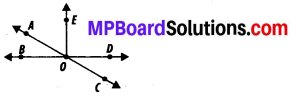(i) Obtuse vertically opposite angles